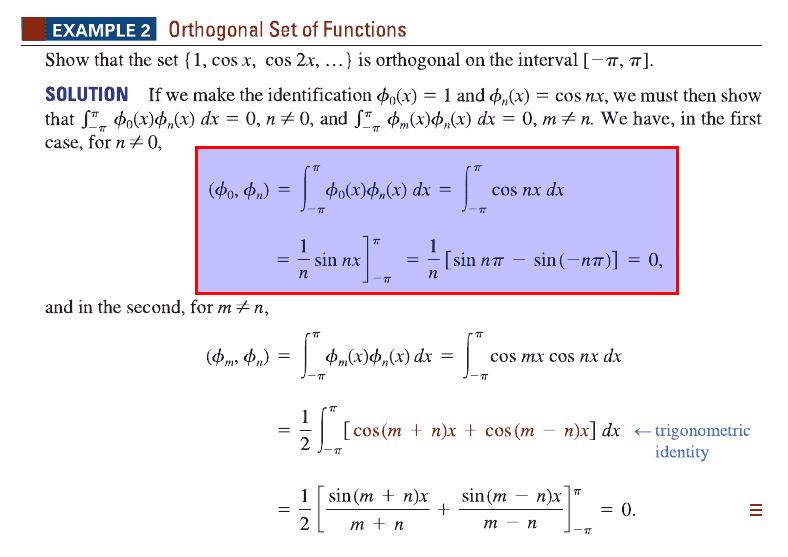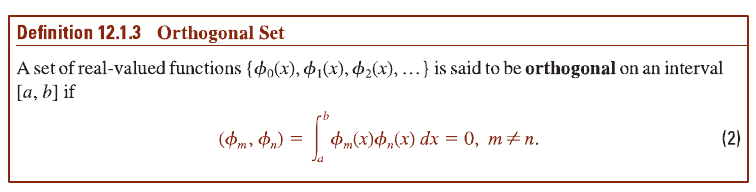# Proving a set of functions is orthogonal

ainster31Why is the math in the red box necessary? According to this definition, it isn't:## Answers and Replies

Homework Helper
hi ainster31!Why is the math in the red box necessary? According to this definition, it isn't:sorry, i don't understand your questionthe red box proves that (φ0, φn) = 0 (for n ≠ 0)

ainster31
hi ainster31!sorry, i don't understand your questionthe red box proves that (φ0, φn) = 0 (for n ≠ 0)

According to definition 12.1.3, a set of real-valued functions can be proven to be orthogonal if (φm, φn) = 0. So why is it necessary to prove (φ0, φn) = 0?

Homework Helper
m=0 is contained as a particular case for arbitrary m and n. It's no need to make the particular case. The proof goes directly by putting cos a = Re (e^ia).

ainster31
m=0 is contained as a particular case for arbitrary m and n. It's no need to make the particular case.

So you're saying it was unnecessary?

The proof goes directly by putting cos a = Re (e^ia).

That went over my head.

Homework Helper
According to definition 12.1.3, a set of real-valued functions can be proven to be orthogonal if (φm, φn) = 0. So why is it necessary to prove (φ0, φn) = 0?

because φo is a member of the set